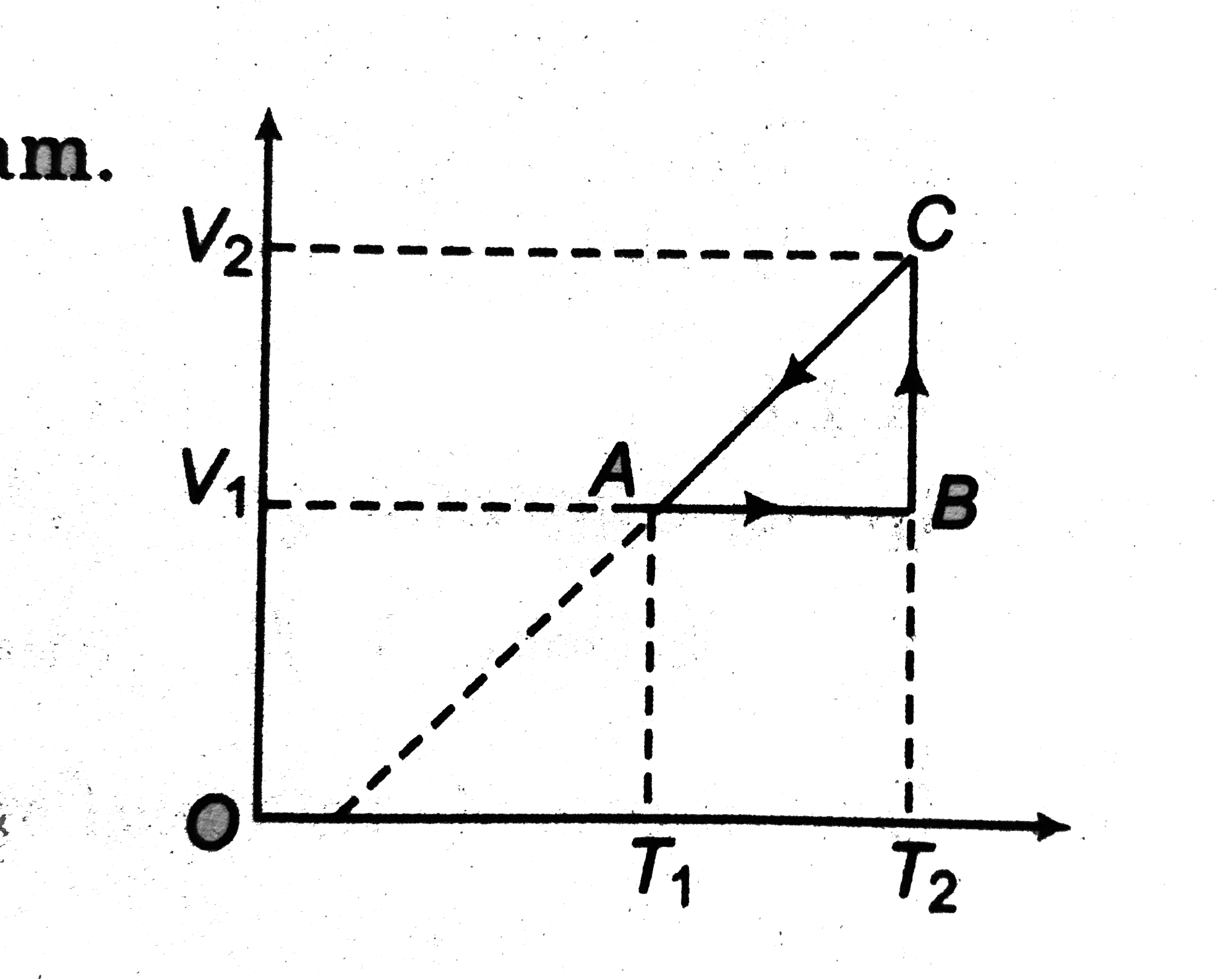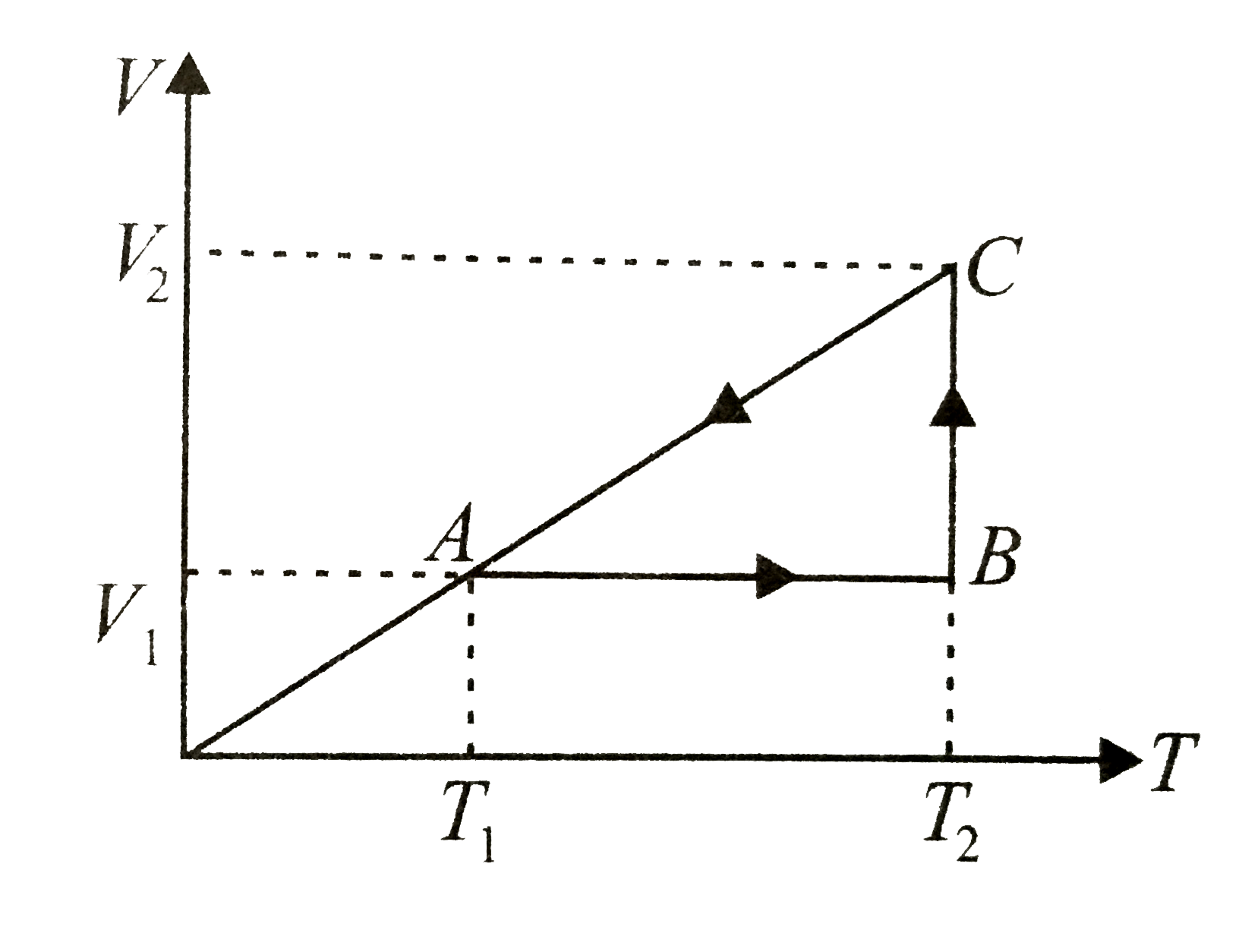# The cyclic process for 1 mole of an ideal gas is shown in the V-T diagram. The work done in AB, BC and CA respectively is

57 views
in Physics
closed
The cyclic process for 1 mole of an ideal gas is shown in the V-T diagram. The work done in AB, BC and CA respectively isA. 0,RT_(1)Ln((V_(1))/(V_(2))),R(T_(1)-T_(2))
B. R,(T_(1)-T_(2))R,RT_(1)In(V_(1)/(V_(2)))
C. 0,RT_(2)ln((V_(2))/(V_(1))),(RT_(1))/(V_(1))(V_(1)-V_(2))
D. 0,RT_(2)ln(V_(1))/(V_(2)),R(T_(1)-T_(2))

by (86.1k points)
selected

During AB, process is isochoric
therefore DeltaV=0
thereforeW=0
During BC, process is
isothermal
therefore DeltaT=0
therefore W=RT_(2)ln(V_(2)/(V_(1)))During CA, process is isobarci. So pressure is constant
therefore W=P(V_(1)-V_(2))
But PV_(1)=rT_(1) therefore P=(RT_(1))/(V_(1))=(RT_(2))/(V_(2))
therefore W=(RT_(1))/(V_(1))(V_(1)-V__(2))=(RT_(2))/(V_(2))(V_(1)-V_(2))